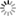##### Personal tools
You are here: Home Linear Algebra

# Linear Algebra

### - Overview

Linear algebra is the branch of mathematics concerning linear equations. Linear algebra is central to almost all areas of mathematics. Linear algebra is the study of linear sets of equations and their transformation properties. Linear algebra allows the analysis of rotations in space, least squares fitting, solution of coupled differential equations, determination of a circle passing through three given points, as well as many other problems in mathematics, physics, and engineering. Confusingly, linear algebra is not actually an algebra in the technical sense of the word "algebra" (i.e., a vector space  over a field , and so on).

Linear algebra, mathematical discipline that deals with vectors and matrices and, more generally, with vector spaces and linear transformations. Unlike other parts of mathematics that are frequently invigorated by new ideas and unsolved problems, linear algebra is very well understood. Its value lies in its many applications, from mathematical physics to modern algebra and coding theory.

Linear algebra is a field of mathematics that is universally agreed to be a prerequisite to a deeper understanding of machine learning. Although linear algebra is a large field with many esoteric theories and findings, the nuts and bolts tools and notations taken from the field are practical for machine learning practitioners. Linear algebra is the mathematics of data. It has had a marked impact on the field of statistics. Linear algebra underlies many practical mathematical tools, such as Fourier series and computer graphics.

### - Linear Algebra Topics

The linear algebra prerequisite should include the following topics:

• Mathematical operations with matrices (addition, multiplication)
• Matrix inverses and determinants
• Solving systems of equations with matrices
• Euclidean vector spaces
• Eigenvalues and eigenvectors
• Orthogonal matrices
• Positive definite matrices
• Linear transformations
• Projections
• Linear dependence and independence
• Singular value decomposition

### - Linear Algebra for Data Science and Machine Learning

Linear Algebra is a continuous form of mathematics and is applied throughout science and engineering because it allows you to model natural phenomena and to compute them efficiently. Because it is a form of continuous and not discrete mathematics, a lot of computer scientists don’t have a lot of experience with it. Linear Algebra is also central to almost all areas of mathematics like geometry and functional analysis. Its concepts are a crucial prerequisite for understanding the theory behind Machine Learning, especially if you are working with Deep Learning Algorithms. You don’t need to understand Linear Algebra before getting started with Machine Learning, but at some point, you may want to gain a better understanding of how the different Machine Learning algorithms really work under the hood. This will help you to make better decisions during a Machine Learning system’s development. So if you really want to be a professional in this field, you will have to master the parts of Linear Algebra that are important for Machine Learning.

In Linear Algebra, data is represented by linear equations, which are presented in the form of matrices and vectors. Therefore, you are mostly dealing with matrices and vectors rather than with scalars. When you have the right libraries, like Numpy, at your disposal, you can compute complex matrix multiplication very easily with just a few lines of code.

[More to come ...]

##### Document Actions